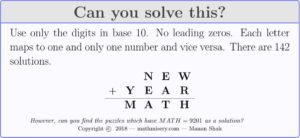# Ten New Year’s Algebra Puzzles For 2019

Alright, the holidays are coming to an end and the second half of the school year is about to begin. Let’s work off some of the rust! Here are a few puzzles to give to your students as warm-ups or challenges depending on where they are.

### Puzzle #1

Find all quadratics such that solutions to $$(ax + b)(cx + d) = 0$$ are integer with $$a,b,c,d$$ all unique and chosen only from $$\{2,0,1,9\}$$

Some gotchas and things to note:

• $$(0x + 2)(1x + 9)$$ isn’t a quadratic! Or at the least, you can have a nice debate about this.
• I leave it to you to decide if you will accept $$(2x + 9)(1x + 0)$$ as a quadratic that satisfies the question above (one of the roots is integer, but the second isn’t).
• Your students be warned: $$(2x + 0)(1x + 9)$$ is the same as $$(1x + 9)(2x + 0)$$!

### Puzzle #2

How many distinct quadratics can be made of the form $$(ax + b)(cx + d)$$ where $$a,b,c,d$$ are all unique and chosen only from $$\{2,0,1,9\}$$?

The answer isn’t $$4! = 24$$ nor is the answer $$\binom{4}{2} = 12$$.

### Puzzle #3

This one depends on Puzzle #2.

If $$a,b,c,d$$ were chosen uniformly randomly without replacement from $$\{2,0,1,9\}$$ what is the probability that $$(ax + b)(cx + d)$$ is a quadratic? What if $$a,b,c,d$$ were chosen uniformly randomly with replacement?

### Puzzle #4

Without using a calculator determine which is larger, $$201^{9}$$ or $$20^{19}$$.

### Puzzle #5

Without using a calculator determine which is larger, $$2^{910}$$ or $$9^{210}$$.

### Puzzle #6

Only using the digits $$2,0,1,9$$ exactly once and assigning them to $$a,b,c,d$$ what’s the largest number that can be formed when written as $$a^{bcd}$$ or $$ab^{cd}$$ or $$abc^{d}$$?

Some points of clarification if there is a sense of ambiguity:

• You can only construct numbers of the three forms given above. The task is to find the construction that will yield the largest value.
• When I write “$$abc$$” I mean digit concatentation, not multiplication. So, if $$a = 2, b = 0, c = 9$$ then $$abc = 209$$

### Puzzle #7

Got fraction troubles? Try this

Choose $$a,b,c,d$$ uniquely from $$\{2,0,1,9\}$$. Which subtraction(s) of the form $$\displaystyle \Big|\frac{a}{b} – \frac{c}{d}\Big|$$ is (are) closest to ONE?

### Puzzle #8

How many four-digit prime numbers can be created using $$\{2,0,1,9\}$$ exactly once? And what are they, if there are any? No calculator allowed!

### Puzzle #9

Try this alphanumeric puzzle for the new year!### Puzzle #10

Construct two lines, one with positive slope and one with negative slope, such that their intersection is at the point $$(20,19)$$.

#### A request!

Thank you for reading! I want to keep in touch with my readers. If you are interested, please have a look here.

Do you enjoy this blog?
Consider supporting with a contribution!## 3 thoughts on “Ten New Year’s Algebra Puzzles For 2019”

1.Adil Mohammed

please send soluton

1.Adil Mohammed

Puzzle 1# Quadratics Equations are (1x+2) (9x+0), (1x+9) (2x+0)

1.Manan Shah Post author

nicely done! Those certainly work.